import pandas as pd
import numpy as np
import matplotlib.pyplot as plt
import seaborn as sns

plt.rcParams['figure.figsize'] = (10, 5)
plt.style.use('fivethirtyeight')


## Working with more than one time series

Whether it is during personal projects or your day-to-day work as a Data Scientist, it is likely that you will encounter situations that require the analysis and visualization of multiple time series at the same time.

Provided that the data for each time series is stored in distinct columns of a file, the pandas library makes it easy to work with multiple time series. In the following exercises, you will work with a new time series dataset that contains the amount of different types of meat produced in the USA between 1944 and 2012.

meat = pd.read_csv('./dataset/ch4_meat.csv')

# Review the first five lines of the meat DataFrame

# Convert the date column to a datestamp type
meat['date'] = pd.to_datetime(meat['date'])

# Set the date column as the index of your DataFrame meat
meat = meat.set_index('date')

# Print the summary statistics of the DataFrame
print(meat.describe())

         date   beef   veal    pork  lamb_and_mutton  broilers  other_chicken  \
0  1944-01-01  751.0   85.0  1280.0             89.0       NaN            NaN
1  1944-02-01  713.0   77.0  1169.0             72.0       NaN            NaN
2  1944-03-01  741.0   90.0  1128.0             75.0       NaN            NaN
3  1944-04-01  650.0   89.0   978.0             66.0       NaN            NaN
4  1944-05-01  681.0  106.0  1029.0             78.0       NaN            NaN

turkey
0     NaN
1     NaN
2     NaN
3     NaN
4     NaN
beef        veal         pork  lamb_and_mutton     broilers  \
count   827.000000  827.000000   827.000000       827.000000   635.000000
mean   1683.463362   54.198549  1211.683797        38.360701  1516.582520
std     501.698480   39.062804   371.311802        19.624340   963.012101
min     366.000000    8.800000   124.000000        10.900000   250.900000
25%    1231.500000   24.000000   934.500000        23.000000   636.350000
50%    1853.000000   40.000000  1156.000000        31.000000  1211.300000
75%    2070.000000   79.000000  1466.000000        55.000000  2426.650000
max    2512.000000  215.000000  2210.400000       109.000000  3383.800000

other_chicken      turkey
count     143.000000  635.000000
mean       43.033566  292.814646
std         3.867141  162.482638
min        32.300000   12.400000
25%        40.200000  154.150000
50%        43.400000  278.300000
75%        45.650000  449.150000
max        51.100000  585.100000


### Visualize multiple time series

If there are multiple time series in a single DataFrame, you can still use the .plot() method to plot a line chart of all the time series. Another interesting way to plot these is to use area charts. Area charts are commonly used when dealing with multiple time series, and can be used to display cumulated totals.

With the pandas library, you can simply leverage the .plot.area() method to produce area charts of the time series data in your DataFrame.

ax = meat.plot(linewidth=2, fontsize=12);

ax.set_xlabel('Date');
ax.legend(fontsize=12);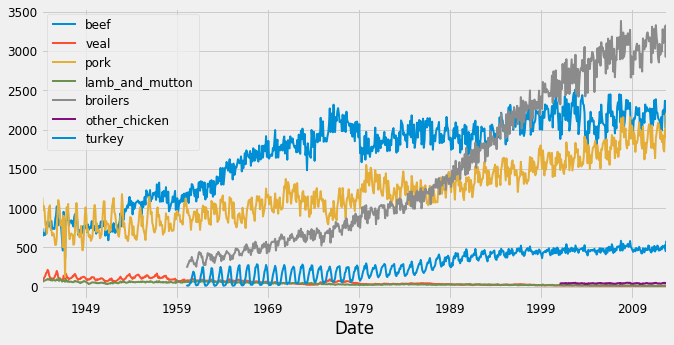ax = meat.plot.area(fontsize=12);

ax.set_xlabel('Date');
ax.legend(fontsize=12);## Plot multiple time series

### Define the color palette of your plots

When visualizing multiple time series, it can be difficult to differentiate between various colors in the default color scheme.

To remedy this, you can define each color manually, but this may be time-consuming. Fortunately, it is possible to leverage the colormap argument to .plot() to automatically assign specific color palettes with varying contrasts. You can either provide a matplotlib colormap as an input to this parameter, or provide one of the default strings that is available in the colormap() function available in matplotlib (all of which are available here).

For example, you can specify the 'viridis' colormap using the following command:

df.plot(colormap='viridis')

ax = meat.plot(colormap='cubehelix', fontsize=15);

ax.set_xlabel('Date');
ax.legend(fontsize=12);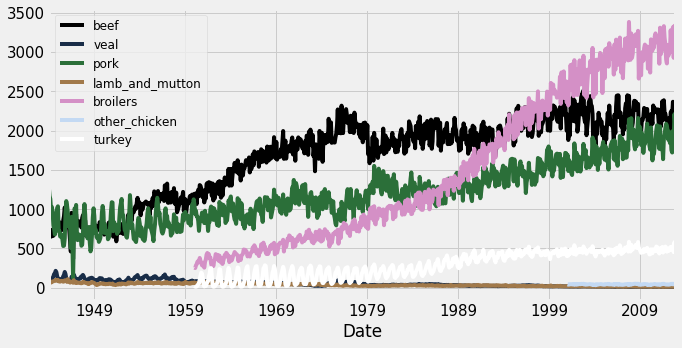ax = meat.plot(colormap='PuOr', fontsize=15);

ax.set_xlabel('Date');
ax.legend(fontsize=12);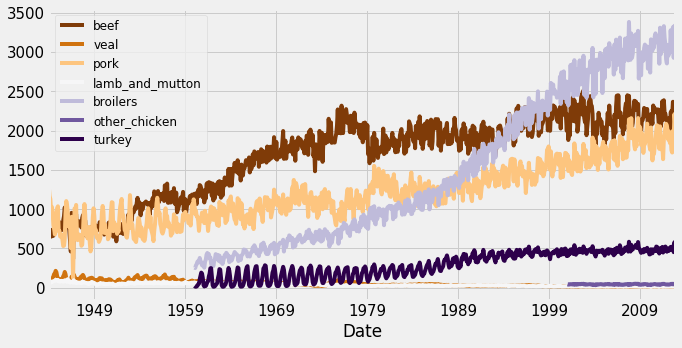It is possible to visualize time series plots and numerical summaries on one single graph by using the pandas API to matplotlib along with the table method:

# Plot the time series data in the DataFrame
ax = df.plot()

# Compute summary statistics of the df DataFrame
df_summary = df.describe()

# Add summary table information to the plot
ax.table(cellText=df_summary.values,
colWidths=[0.3]*len(df.columns),
rowLabels=df_summary.index,
colLabels=df_summary.columns,
loc='top')

meat_mean = meat.mean().to_frame().T
meat_mean.rename({0:'mean'}, inplace=True)

meat_mean

beef veal pork lamb_and_mutton broilers other_chicken turkey
mean 1683.463362 54.198549 1211.683797 38.360701 1516.58252 43.033566 292.814646
ax = meat.plot(fontsize=10, linewidth=1);

ax.set_xlabel('Date', fontsize=10);

# Add summary table information to the plot
ax.table(cellText=meat_mean.values,
colWidths=[0.15] * len(meat_mean.columns),
rowLabels=meat_mean.index,
colLabels=meat_mean.columns,
loc='top');

# Specify the fontsize and location of your legend
ax.legend(loc='upper center', bbox_to_anchor=(0.5, 0.95), ncol=3, fontsize=10);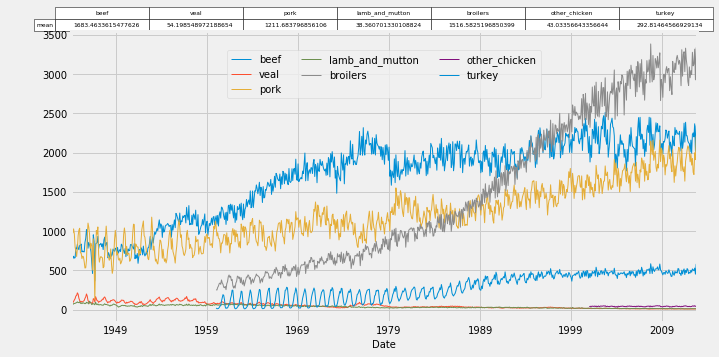### Plot your time series on individual plots

It can be beneficial to plot individual time series on separate graphs as this may improve clarity and provide more context around each time series in your DataFrame.

It is possible to create a "grid" of individual graphs by "faceting" each time series by setting the subplots argument to True. In addition, the arguments that can be added are:

• layout: specifies the number of rows x columns to use.
• sharex and sharey: specifies whether the x-axis and y-axis values should be shared between your plots.
meat.plot(subplots=True,
layout=(2, 4),
sharex=False,
sharey=False,
colormap='viridis',
fontsize=8,
legend=False,
linewidth=0.2);
plt.tight_layout();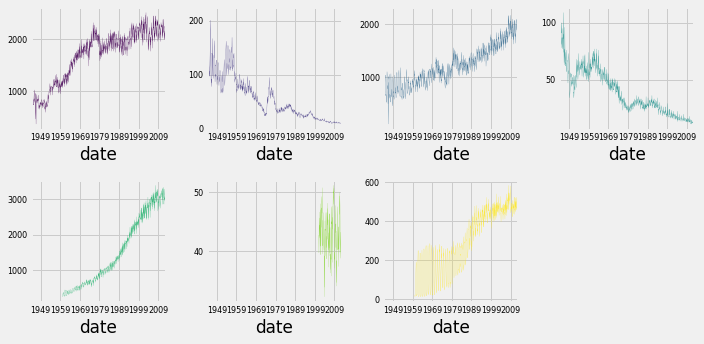## Find relationships between multiple time series

• Correlations between two variables
• In the field of Statistics, the correlation coefficient is a measure used to determine the strength or lack of relationship between two variables:
• Pearson's coefficient can be used to compute the correlation coefficient between variables for which the relationship is thought to be linear
• Kendall Tau or Spearman rank can be used to compute the correlation coefficient between variables for which the relationship is thought to be non-linear
• What is a correlation matrix?
• When computing the correlation coefficient between more than two variables, you obtain a correlation matrix
• Range: [-1, 1]
• 0: No relationship
• 1: Strong positive relationship
• -1: Strong negative relationship
• A correlation matrix is always "symmetric"
• The diagonal values will always be equal to 1

### Compute correlations between time series

The correlation coefficient can be used to determine how multiple variables (or a group of time series) are associated with one another. The result is a correlation matrix that describes the correlation between time series. Note that the diagonal values in a correlation matrix will always be 1, since a time series will always be perfectly correlated with itself.

Correlation coefficients can be computed with the pearson, kendall and spearman methods. A full discussion of these different methods is outside the scope of this course, but the pearson method should be used when relationships between your variables are thought to be linear, while the kendall and spearman methods should be used when relationships between your variables are thought to be non-linear.

print(meat[['beef', 'pork']].corr(method='spearman'))

# Print the correlation between beef and port columns

          beef      pork
beef  1.000000  0.827587
pork  0.827587  1.000000

print(meat[['pork', 'veal', 'turkey']].corr(method='pearson'))

            pork      veal    turkey
pork    1.000000 -0.808834  0.835215
veal   -0.808834  1.000000 -0.768366
turkey  0.835215 -0.768366  1.000000


### Visualize correlation matrices

The correlation matrix generated in the previous exercise can be plotted using a heatmap. To do so, you can leverage the heatmap() function from the seaborn library which contains several arguments to tailor the look of your heatmap.

df_corr = df.corr()

sns.heatmap(df_corr)
plt.xticks(rotation=90)
plt.yticks(rotation=0)


You can use the .xticks() and .yticks() methods to rotate the axis labels so they don't overlap.

To learn about the arguments to the heatmap() function, refer to this page.

corr_meat = meat.corr(method='spearman')

# Customize the heatmap of the corr_meat correlation matrix
sns.heatmap(corr_meat,
annot=True,
linewidths=0.4,
annot_kws={'size': 10});

plt.xticks(rotation=90);
plt.yticks(rotation=0);### Clustered heatmaps

Heatmaps are extremely useful to visualize a correlation matrix, but clustermaps are better. A Clustermap allows to uncover structure in a correlation matrix by producing a hierarchically-clustered heatmap:

pyrhon
df_corr = df.corr()

fig = sns.clustermap(df_corr)
plt.setp(fig.ax_heatmap.xaxis.get_majorticklabels(), rotation=90)
plt.setp(fig.ax_heatmap.yaxis.get_majorticklabels(), rotation=0)

To prevent overlapping of axis labels, you can reference the Axes from the underlying fig object and specify the rotation. You can learn about the arguments to the clustermap() function here.

corr_meat = meat.corr(method='pearson')

# Customize the heatmap of the corr_meat correlation matrix
fig = sns.clustermap(corr_meat,
row_cluster=True,
col_cluster=True,
figsize=(10, 10));

plt.setp(fig.ax_heatmap.xaxis.get_majorticklabels(), rotation=90);
plt.setp(fig.ax_heatmap.yaxis.get_majorticklabels(), rotation=0);
plt.savefig('../images/clustermap.png')